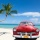Home Communities
IT Knowledge
Inspiration
Languages
EN

# JavaScript - how to sort array?

14 points
Created by:Jan-Alfaro
351

## 1. Overview

In JavaScript it is possible to sort array in following ways.

## 2. Sorting array in ascending ordering

In this example numbers are sorted from smallest to biggest - `Array` `sort` (`Array.prototype.sort`) method with ascending ordering has been used.

``````var array = [5, 2, 3, 1, 4];
array.sort();

console.log(array);``````

Output:

``[ 1, 2, 3, 4, 5 ]``

## 3. Sorting array in ascending ordering with compare function

In this example numbers are sorted from smallest to biggest with compare function.

``````var array = [5, 2, 3, 1, 4];

array.sort(function(a, b) {
return a - b;
});

console.log(array);``````

Output:

``[ 1, 2, 3, 4, 5 ]``

## 4. Sorting array in desscending ordering with compare function

In this example numbers are sorted from biggest to smallest - in this case compare function is necessary.

``````var array = [5, 2, 3, 1, 4];

array.sort(function(a, b) {
return b - a;
});

console.log(array);``````

Output:

``[ 5, 4, 3, 2, 1 ]``

## 5. Sorting array in desscending ordering with reverse method example

In this example numbers are sorted from biggest to smallest - in this case reverse function has been used.

``````var array = [5, 2, 3, 1, 4];

var result = array.sort()
.reverse();

console.log(result);``````

Output:

``[ 5, 4, 3, 2, 1 ]``